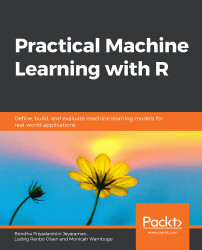•#### Practical Machine Learning with R#### Overview of this book

With huge amounts of data being generated every moment, businesses need applications that apply complex mathematical calculations to data repeatedly and at speed. With machine learning techniques and R, you can easily develop these kinds of applications in an efficient way. Practical Machine Learning with R begins by helping you grasp the basics of machine learning methods, while also highlighting how and why they work. You will understand how to get these algorithms to work in practice, rather than focusing on mathematical derivations. As you progress from one chapter to another, you will gain hands-on experience of building a machine learning solution in R. Next, using R packages such as rpart, random forest, and multiple imputation by chained equations (MICE), you will learn to implement algorithms including neural net classifier, decision trees, and linear and non-linear regression. As you progress through the book, you’ll delve into various machine learning techniques for both supervised and unsupervised learning approaches. In addition to this, you’ll gain insights into partitioning the datasets and mechanisms to evaluate the results from each model and be able to compare them. By the end of this book, you will have gained expertise in solving your business problems, starting by forming a good problem statement, selecting the most appropriate model to solve your problem, and then ensuring that you do not overtrain it.Free Chapter
An Introduction to Machine LearningData Cleaning and Pre-processingFeature EngineeringIntroduction to neuralnet and Evaluation MethodsLinear and Logistic Regression ModelsUnsupervised LearningAppendix## Classification

The goal of classification is to create a model that can predict classes in never-before-seen data. This means that the model should generalize beyond the training data. As the data we work with is supervised, meaning that we already know the answer to our question (does the applicant have a good or bad credit rating?), it is rarely interesting to have a model that can merely repeat that. Instead, we need the model to classify the unlabeled data we gather in the future. We will discuss this further when covering under- and overfitting.

The datasets being used are the following:

### Binary Classification

In binary classification, our question is of the either or type. There are only two options, either yes or no; not maybe and not both yes and no. In multiclass classification, we can have more than two options, though we can only choose one of them. In multilabel classification, it is possible to predict both yes and no at the same time, hence an...